### CAT 1990 Question Paper Question 70

Instructions

The first table gives the number of saris (of all the eight colours) stocked in six regional showrooms. The second gives the number of saris (of all the eight colours) sold in these six regional showrooms. The third table gives the percentage of saris sold to saris stocked for each colour in each region. The fourth table gives the percentage of saris of a specific colour sold within that region. The fifth table gives the percentage of saris of a specific colour sold across all the regions. Study the tables and for each of the following questions, choose the best alternative.

Table 1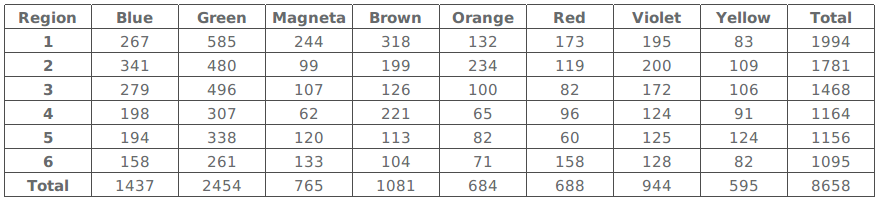Table 2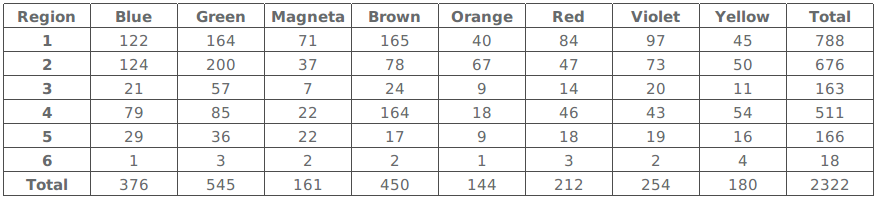Table 3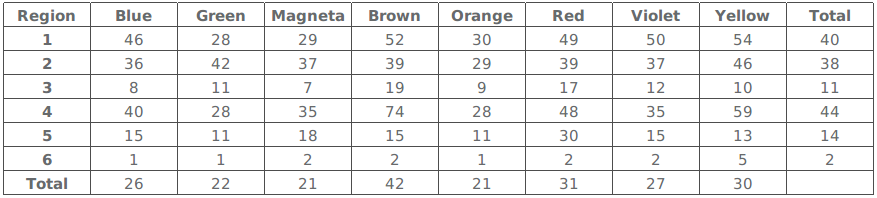Table 4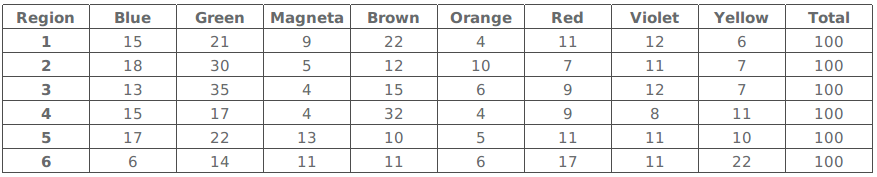Table 5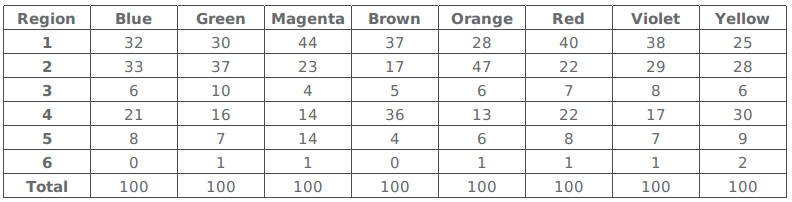Question 70

# Out of its total sales, which region sold the minimum percentage of green saris?

Solution

Given we have 6 regions where sales are defined in the table number two . Now given that we need to find the region where green saris as a percentage of the total sales is lowest . In order to do that we calculate Green saris sold in a region/ Total sales of a particular region by considering the values from table 2 .

Now for option 1 which is region 1 the value is (164/788)*100  = 20.81 %

For option 2 which is region 4 the value is (85/511)*100 = 16.63 %

For option 1 which is region 6 the value is (3/18)*100 = 16.66 %

For option 1 which is region 2  the value is (200/676)*100 = 29.58  % .

Hence among the given options the percentage of region 4 is lowest which is option 2 .

• All Quant CAT Formulas and shortcuts PDF
• 30+ CAT previous papers with solutions PDF